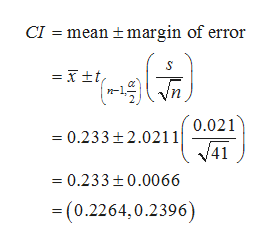# That is a typical measurement that is taken when judging concussions as well as looking at motor skills. At the beginning of the study they recorded a baseline average reaction time for the group of 41 football players at .239 seconds. At the end of their study they retested the players and the average reaction time was .233 with a standard deviation of .021. Use this data to create a 95% confidence interval for µ. Round to four decimal places. (-0.187,0.653)Question:Based on your confidence interval, explain if the reaction time at the conclusion of the study showed a significant decrease in the reaction time or not.

Question
1 views

That is a typical measurement that is taken when judging concussions as well as looking at motor skills. At the beginning of the study they recorded a baseline average reaction time for the group of 41 football players at .239 seconds. At the end of their study they retested the players and the average reaction time was .233 with a standard deviation of .021. Use this data to create a 95% confidence interval for µ. Round to four decimal places. (-0.187,0.653)

Question:Based on your confidence interval, explain if the reaction time at the conclusion of the study showed a significant decrease in the reaction time or not.

check_circle

Step 1

Calculating the confidence interval for population mean:

Sample mean = 0.233 seconds.

Sample standard deviation, s is 0.021 seconds.

The sample size, n is 41.

From the EXCEL, usi...help_outlineImage TranscriptioncloseCI = mean + margin of error = I±t, 0.021 = 0.233+2.0211 41 = 0.233+0.0066 = (0.2264,0.2396) fullscreen

### Want to see the full answer?

See Solution

#### Want to see this answer and more?

Solutions are written by subject experts who are available 24/7. Questions are typically answered within 1 hour.*

See Solution
*Response times may vary by subject and question.
Tagged in

### Other